# Long Answers - Gravitation, Science, Class 9 Notes | Study Class 9 Science by VP Classes - Class 9

## Class 9: Long Answers - Gravitation, Science, Class 9 Notes | Study Class 9 Science by VP Classes - Class 9

The document Long Answers - Gravitation, Science, Class 9 Notes | Study Class 9 Science by VP Classes - Class 9 is a part of the Class 9 Course Class 9 Science by VP Classes.
All you need of Class 9 at this link: Class 9

Q1. Show that
(a) the acceleration due to gravity at the surface of moon is about one-sixth of that at the surface of the earth.
(b) the weight of the body on moon = 1/6 th the weight of the body on earth.
Ans.
(a) The acceleration due to gravity ‘g’ is given by the formula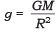G = 6.67 × 10−11

 celestial Body Mass (kg) Radius (m) Earth 5.98 x 1024 6.37 x 106 Moon 7.36 x 1022 1.74 x 106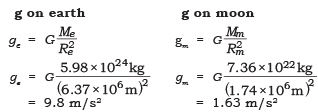The value of g on moon is 1.63 m/s
The value of g on earth is 9.8 m/s
∴ g on moon is (1/6)th g on earth.

(b) Weight of the body is given by formula W = m × g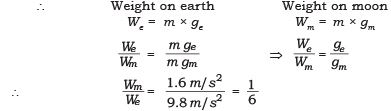∴    Weight of the body on moon = (1/6) th the weight of the body on earth.

Q2. The acceleration of a freely falling body does not depend on the mass of the body. Show this by deriving an expression for the same.
Ans.
Newton’s law of gravitation states that every two bodies in the universe attract each other with a force which is directly proportional to the product of their masses and inversely proportional to the square of distance between them.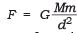...(i)

Newton’s second law of motion shows that force is the product of mass and acceleration. Let m be the mass of the body, the gravitational force causes acceleration in it, is denoted by ‘g’

∴      F = mg                 ...(ii)
From (i) and (ii) we get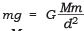∵ M = mass of the earth
d = distance between the object and the earth
Let the object be on the surface of the earth. The distance d = R (radius of the earth).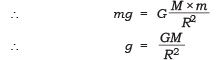Q3. Establish a relationship between ‘g’ and ‘G’.
Ans.
‘g’ is acceleration due to gravity.
The gravitational force ‘F’ is exerted on a body of mass ‘m’, the body gets accelerated ‘g’
∴                F = mg     ...(i)
‘G’ is the universal gravitational constant. Newton’s law of universal gravitational constant gives the formula

F =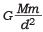...(ii)
when M = Mass of earth
m = mass of object
d = distance between the two bodies
From equation (i) and (ii) we get

mg =For a body on the surface of earth d = R (radius of the earth)
∴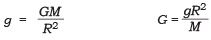Q4. With the help of an activity prove that the force acting on a smaller area exerts a larger pressure?
Ans.
Consider a block of wood kept on a table top. The mass of the wooden block is 5 kg. Its dimension is 40 cm × 20 cm × 10 cm.
Now, we have to find the pressure exerted by the wooden block on the table top by keeping it vertically and horizontally.
The mass of the wooden block = 5 kg Weight of the wooden block applies a thrust on the table top
∴ Thrust = F = m × g = 5 kg × 9.8 m/s2 = 49 N
(case a)—When the wooden box is kept vertically with sides 20 cm × 10 cm.
Area of a side = length × breadth
= 20 cm × 10 cm
= 200 cm= 0.02 m2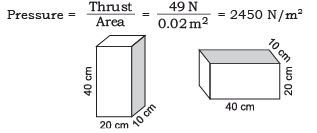(case b)—When the block is kept horizontally with side 40 cm × 20 cm.
= 40 cm × 20 cm
= 800 cm2 = 0.08 m2

⇒  Pressure = Thrust / Area

= 49 N/ 0.08 m2  = 612.5 N/m2

∴ The pressure exerted by the box in case

(a) is more as compared to the pressure exerted in case
(b).The area is reduced and the pressure exerted is more.
This shows that pressure ∝ (1 /area)

Pressure will be larger if the area is reduced.

Application:

• Nails have pointed tips.
• Knives have sharp edges.
• Needles have pointed tips.

Q5. Calculate the gravitational force of a planet of mass 4.5 × 109 kg on a moon of mass 2.2 × 108 kg, if the distance between their centres is 6.8 × 107 m. Calculate the acceleration due to gravity on the surface of the given planet, given that it has a radius of 9.0 × 104 m.
Ans.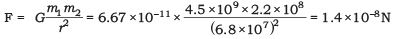The gravitational force is on the moon is 1.4 × 10–8 N (2 s.f.) towards the centre of the planet.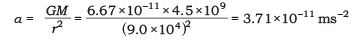The acceleration at the surface of the planet is 3.7 × 10–11 ms–2 (2 s.f.) towards the planet’s centre.

Q6. A buoyant force of 26 N acts on a piece of metal that is completely immersed in ethyl alcohol. What is the volume of the metal piece? The density of ethanol = 785.06 kg/m3.
Ans.
Buoyant force = density x volume x g
26N = 785.06 × V × 9.8
V = 3379.5 cm3

Q7. Find buoyant force on the lead block. Weight of lead block = 1.1 N, Volume of block = 10 cm3. 1 cm3 of water weighs 0.0098 N.
Ans.
The lead displaces 10 cm3 of water.
Buoyant force = weight of water displaced 10 cmof water × 0.0098 N/cm3 = 0.098 N.

The document Long Answers - Gravitation, Science, Class 9 Notes | Study Class 9 Science by VP Classes - Class 9 is a part of the Class 9 Course Class 9 Science by VP Classes.
All you need of Class 9 at this link: Class 9Use Code STAYHOME200 and get INR 200 additional OFF

## Class 9 Science by VP Classes

75 docs|14 tests

Track your progress, build streaks, highlight & save important lessons and more!

,

,

,

,

,

,

,

,

,

,

,

,

,

,

,

,

,

,

,

,

,

,

,

,

,

,

,

;